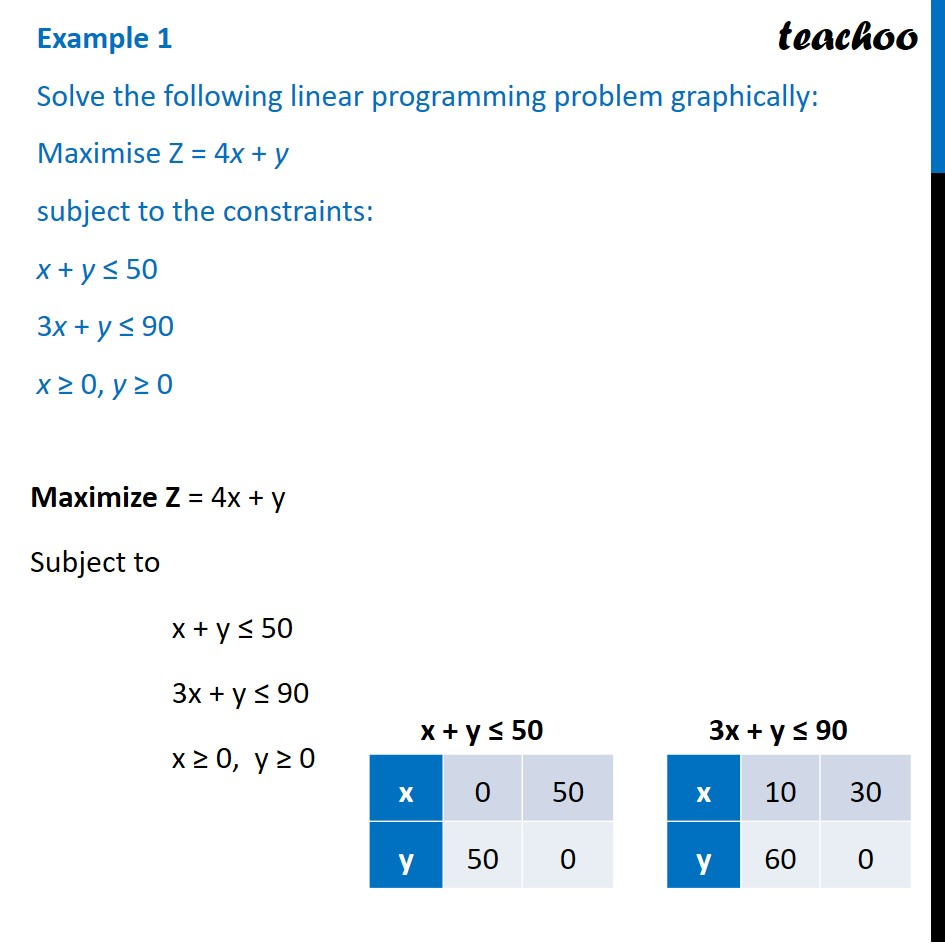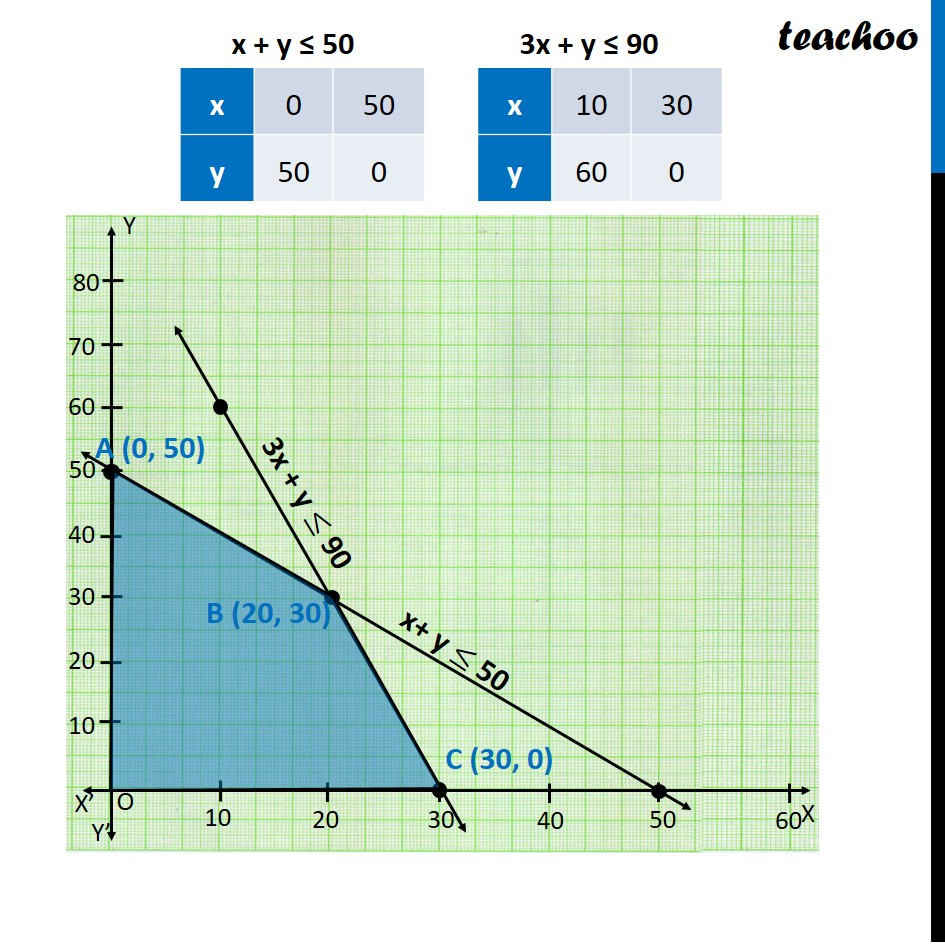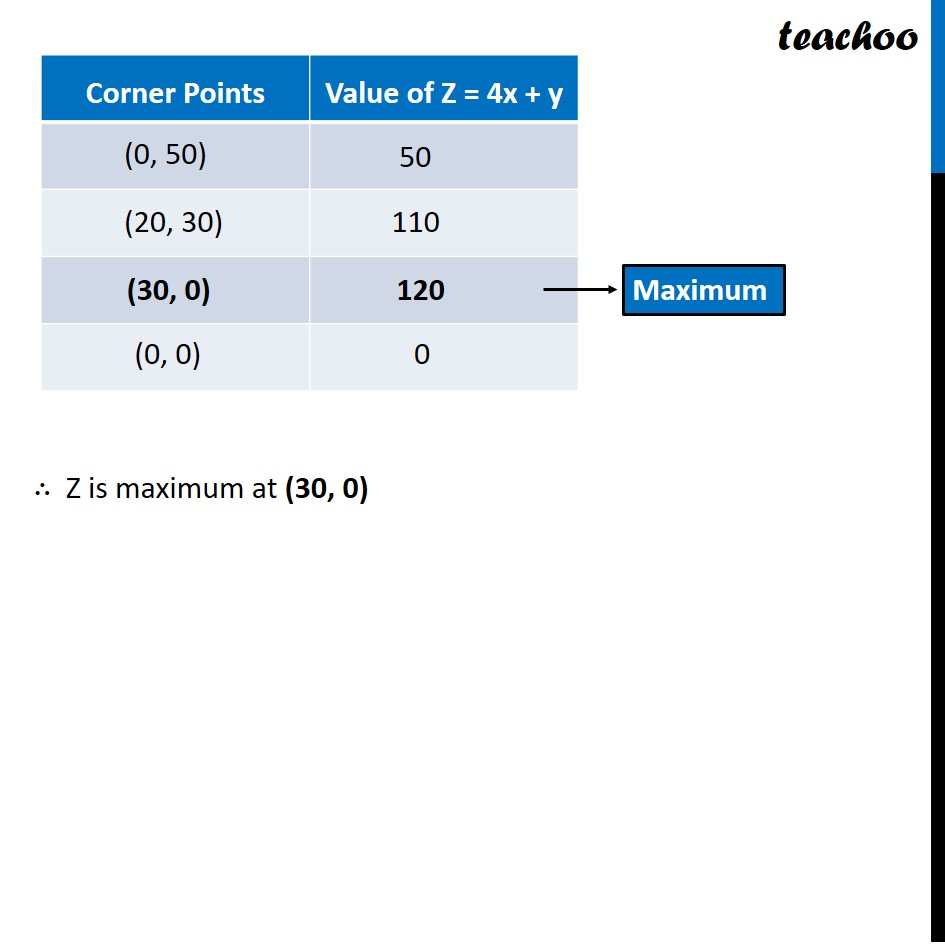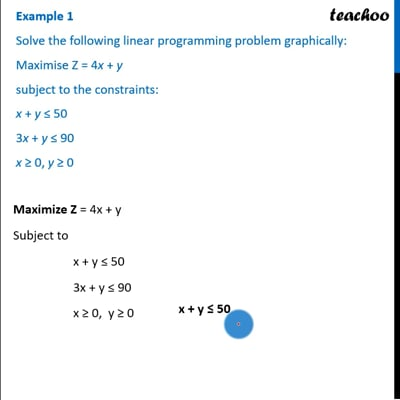Linear equations given - Bounded

Chapter 12 Class 12 Linear Programming
Concept wiseThis video is only available for Teachoo black users

Introducing your new favourite teacher - Teachoo Black, at only ₹83 per month

### Transcript

Example 1 Solve the following linear programming problem graphically: Maximise Z = 4x + y subject to the constraints: x + y ≤ 50 3x + y ≤ 90 x ≥ 0, y ≥ 0 Maximize Z = 4x + y Subject to x + y ≤ 50 3x + y ≤ 90 x ≥ 0, y ≥ 0 ∴ Z is maximum at (30, 0)